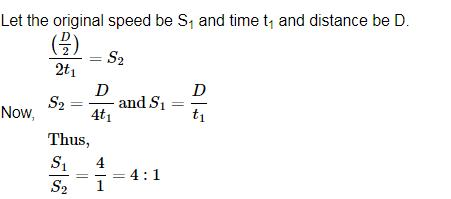Courses

# Time And Distance MCQ 1

## 20 Questions MCQ Test Quantitative Techniques for CLAT | Time And Distance MCQ 1

Description
This mock test of Time And Distance MCQ 1 for Quant helps you for every Quant entrance exam. This contains 20 Multiple Choice Questions for Quant Time And Distance MCQ 1 (mcq) to study with solutions a complete question bank. The solved questions answers in this Time And Distance MCQ 1 quiz give you a good mix of easy questions and tough questions. Quant students definitely take this Time And Distance MCQ 1 exercise for a better result in the exam. You can find other Time And Distance MCQ 1 extra questions, long questions & short questions for Quant on EduRev as well by searching above.
QUESTION: 1

### Train – A crosses a pole in 25 seconds and another Train B crosses a pole in 1 minute and 15 seconds. Length of train A is half length of train B. what is the respective ratio between the speed of train A and Train – B?

Solution:

If the length of train A be x metre, then length of train B = 2x metre

When a train crosses a pole, it covers a distance equal to its own length.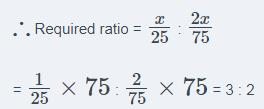QUESTION: 2

### An aeroplane flies with an average speed of 756 km/hr. A helicopter takes 48 hours to cover twice the distance covered by aeroplane in 9 hours. How much distance will the helicopter cover in 18 hours? (assuming that flights are non-stop and moving with uniform speed)

Solution: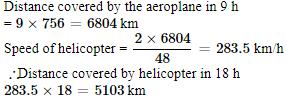QUESTION: 3

### A 280 meters long train travelling at a uniform speed, crosses a platform in 60 seconds and a man standing on the platform in 20 seconds. What is the length of the platform?

Solution: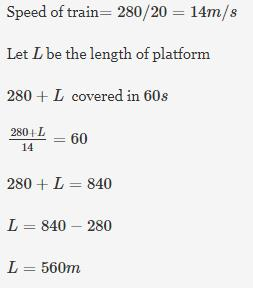QUESTION: 4

A 320 meter long train moving with an average speed of 120 km/hr crosses a platform in 24 seconds. A man crosses the same platform in 4 minutes. What is the speed of man in meter/second?

Solution:

Speed of train = 120 kmph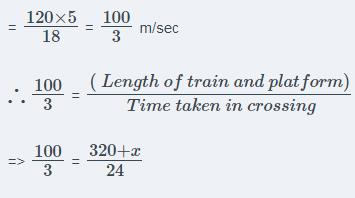Where x = length of platform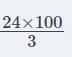= 320 + x

800 = 320 +x

x = 800 - 320 = 480 m

∴ Man's speed =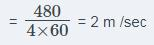QUESTION: 5

A man goes to Mumbai from Pune at a speed of 4 km/hr and returns to Pune at speed of 6km/hr. What is his average speed of the entire journey?

Solution: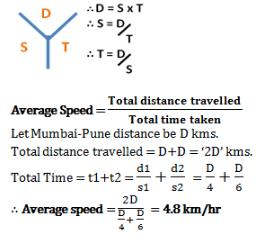QUESTION: 6

A car covers first 39 km of its journey in 45 minutes and the remaining 25 km in 35 minutes. What is the average speed of the car?

Solution: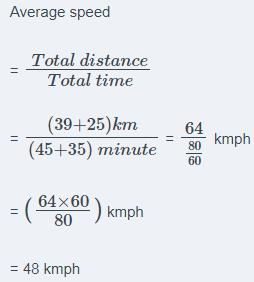QUESTION: 7

Rohit has to reach Mumbai which is 500 km away in 10 hours. His starting speed for 3 hours was 60 km/hr. For the next 200 km his speed was 50km/hr. By what speed he must travel now so as to reach Mumbai in decided time of 10 hours?

Solution: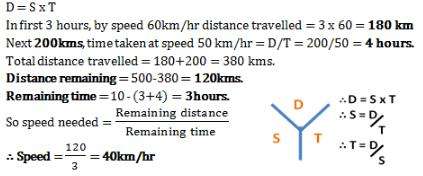QUESTION: 8

Train – A crosses a stationary train –B in 35 seconds and a pole in 14 seconds with the same speed. The length of the train – A is 280 meters. What is the length of the stationary train – B?

Solution:

Let the length of train B be x metre
∴ Speed of train A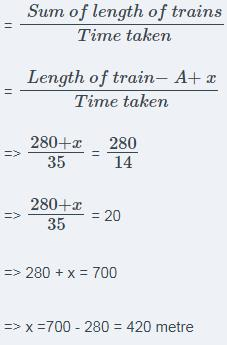QUESTION: 9

A person crosses a 600 m long street in 5 minutes. What is his speed in km per hour?

Solution: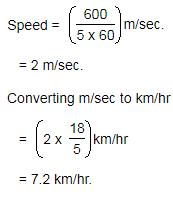QUESTION: 10

A train can travel 50% faster than a car. Both start from point A at the same time and reach point B 75 kms away from A at the same time. On the way, however, the train lost about 12.5 minutes while stopping at the stations. The speed of the car is:

Solution: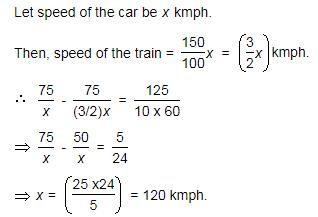QUESTION: 11

In a flight of 600 km, an aircraft was slowed down due to bad weather. Its average speed for the trip was reduced by 200 km/hr and the time of flight increased by 30 minutes. The duration of the flight is:

Solution: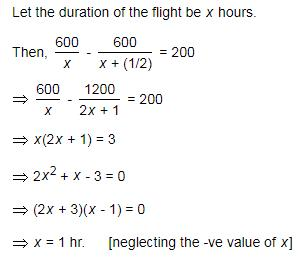QUESTION: 12

A man complete a journey in 10 hours. He travels first half of the journey at the rate of 21 km/hr and second half at the rate of 24 km/hr. Find the total journey in km.

Solution: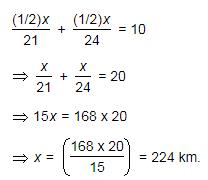QUESTION: 13

A boy goes to school with a speed of 3 km/hr and returns to the village with a speed of 2 km/hr. If he takes 5 hours in all, the distance between the village and the school is

Solution: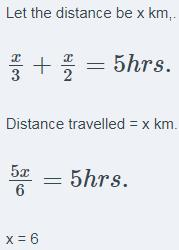QUESTION: 14

Two cyclists A and B start from the same place at the same time, one going towards north at 18 km/hr and other going towards south at 20 km/hr. What time will they take to be 95 km apart?

Solution: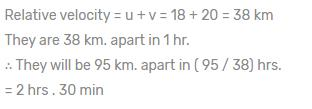QUESTION: 15

Sharad covers two-third of a certain distance at 4 km/hr and the remaining at 5 km/hr. If he takes 42 minutes in all the distance is

Solution: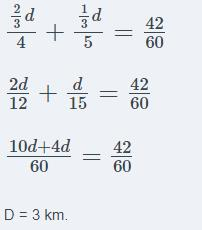QUESTION: 16

A man performs 2/15 of the total journey by rail, 9/20 by tonga and the remaining 10 km on foot. His total journey is

Solution:QUESTION: 17

Raja covers a certain distance in 14 hrs 40 min. He covers one half of the distance by the train at 60 km/hr and the rest half by road at 50 km/hr. The distance traveled by him is

Solution: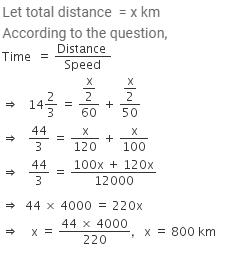QUESTION: 18

Suresh travelled 1200 km by air which formed (2/5) of his trip. One third of the whole trip, he travelled by car and the rest of the journey he performed by train. The distance travelled by trains was

Solution: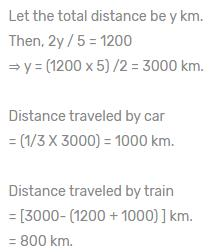QUESTION: 19

If a boy takes as much time in running 10 m as a car takes in covering 25 m, the distance covered by the boy during the time the car covers 1 km is

Solution: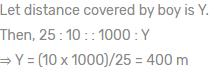QUESTION: 20

A certain distance is covered at a certain speed. If half of this distance is covered in double the time, the ratio of the two speeds is

Solution: